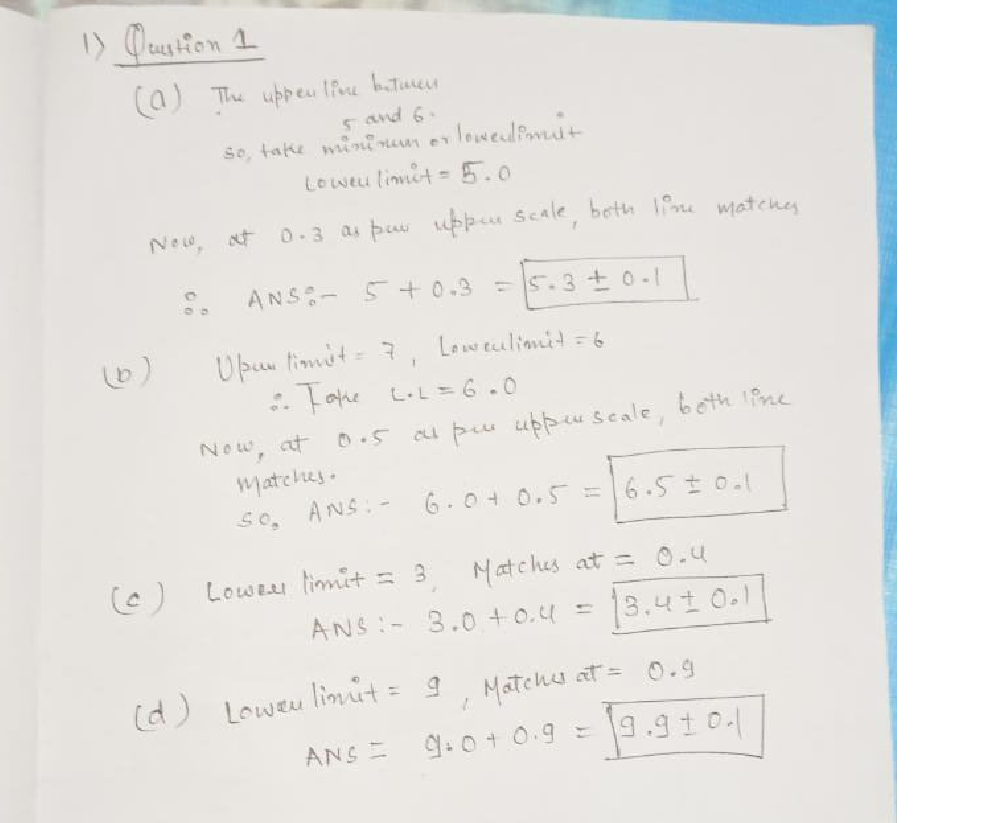Question

Mechanical MeasurementsVerified### Question 45716Mechanical Measurements

A student wants to calculate the volume of a metal tube 3.47±0.05m long. She measures the diameter of the tube in a number of places along its length. Her results (in m) are shown below:
D = 0.48, 0.47, 0.49, 0.48, 0.50, 0.45
a) The percentage uncertainty in the length (I)
b) The mean value of D (in m)
c) The range in measurements of D
d) The absolute uncertainty in D (in m)
e) The percentage uncertainty in D(2 marks)
V=\frac{\pi D^{2} l}{4}
f) The volume of the metal tube using the formula below. State your answer to 2decimal places.(2 marks)
g) The percentage uncertainty in V
h) The absolute uncertainty in V

### Question 45715Mechanical Measurements

### Question 45714Mechanical Measurements

### Question 44572Mechanical Measurements

A student wants to calculate the volume of a metal tube 3.47±0.05m long.She measures the diameter of the tube in a number of places along itslength. Her results (in m) are shown below:
D = 0.48, 0.47, 0.49, 0.48, 0.50, 0.45
a) The percentage uncertainty in the length (1)
b) The mean value of D (in m)
The range in measurements of D
) The absolute uncertainty in D (in m)
The percentage uncertainty in D
O The volume of the metal tube using the formula below. State your answer to 2 decimal places.
) The percentage uncertainty in V
-) The absolute uncertainty in V

### Question 44571Mechanical Measurements

Determine the reading in mm on these micrometer scales. State youranswer with an appropriate uncertainty.

### Question 44570Mechanical Measurements

### Question 40648Mechanical Measurements

A. Consider a plane OA at an angle y with the horizon as shown below. If a projectile is fired up the inclined surface from the point O with an initial velocity v making an angle of with the horizon. The projectile follows a parabolic path and strikes the inclined surface at A.
\text { 1. Derive an expression for the time of flight of the projectile in terms of } v_{n} \gamma \text { and } \theta \text {. }
\text { 2. Derive an expression for the range } \mathrm{R} \text { along the inclined surface in terms of } v, \gamma \text { and } \theta \text {. }
3. Derive an expression for the maximum range Rmax änd the corresponding angle ofprojection.
4. Derive an expression for maximum hight (Hmax) as shown in the figure.

### Question 39406Mechanical Measurements

A mass-spring-damping system is shown in Figure Q4.
Equation (Q4) below describes the input (f(t)) and output (x(t)) function obtained from Newton's law:
f(t)=m \frac{d^{2} x}{d t^{2}}+d \frac{d x}{d t}+k \cdot x(t)
where k = 1kN/m, d = 20N s/m, m= kg
(a) With the given input/output and Equation (Q4), calculate the transfer function.Denote this transfer function as G(s).
(b) Calculate the poles of this system and assess its stability.
(c) Calculate the impulse response of this system. Show details of your calculation.
(d) Calculate the step response for the sampling points given in Table Q4. Show details of calculation.

### Question 39405Mechanical Measurements

For the approximate magnitude Bode plot shown in Figure Q5 below, find:
(a) Corresponding transfer function H(s).
(b) Corresponding approximate Phase Bode plot.

### Question 36438Mechanical Measurements

Measurements were taken as a ball falls a distance D in a time T are:
D = 1.215+ 0.004 m
T = 0.495, 0.498, 0.530, 0.496, 0.501 s
) The mean value of T.
The acceleration due to gravity g, using the formula:
g=\frac{2 D}{T^{2}}
Percentage uncertainty in D.
Range in measurements of T
The percentage uncertainty in the average value of T.
) The absolute uncertainty in the average value of T.
The percentage uncertainty in g.
) The absolute uncertainty in g.

### Submit query